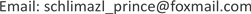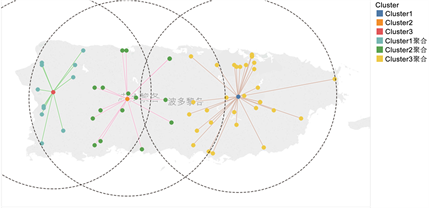﻿ 基于K-Means聚类算法的应急物流中心选址 Location of Emergency Logistics Center Based on K-Means Clustering Algorithm

Pure Mathematics
Vol. 09  No. 07 ( 2019 ), Article ID: 32155 , 4 pages
10.12677/PM.2019.97106

Location of Emergency Logistics Center Based on K-Means Clustering Algorithm

Yujie Guan, Xun Xu, Yajuan Huang, Ye Wu

School of Mathematics and Statistics, Changsha University of Science and Technology, Changsha HunanReceived: Aug. 19th, 2019; accepted: Sep. 9th, 2019; published: Sep. 16th, 2019ABSTRACT

The location of emergency logistics center determines the upper limit of rescue and transportation efficiency. The paper mainly discusses the location of the medicine storage container. Under the background of hurricane attacking Puerto Rico, a series of constraints are selected, and the corresponding mathematical models are established, which are solved by K-means clustering algorithm. Finally, the results of the algorithm are compared with those of fuzzy C clustering algorithm.

Keywords:Location Problem, K-Means Clustering Algorithm, Fuzzy C-Clustering Algorithm1. 引言

2. 问题介绍

2017年，美国的波多黎各(Puerto Rico)遭遇最严重飓风袭击。飓风使该岛遭受严重破坏，造成2900多人死亡。狂风暴雨击倒了波多黎各80％的电线杆和所有输电线路，导致基本上所有岛屿都失去了电力。此外，风暴还破坏或摧毁了岛上大部分的蜂窝通信网络。岛上大部分地区的电力和电池服务中断持续数月，而在某些地区则更长。广泛的洪水阻塞并破坏了岛上的许多高速公路和道路，使得紧急服务地面车辆几乎不可能规划和导航他们的路线。波多黎各的数十个地区被孤立，没有通信。

3. 模型建立以及求解流程 

3.1. 建立选址问题的数学模型

$\mathrm{min}\underset{j=1}{\overset{k}{\sum }}\underset{x\in {s}_{j}}{\sum }{y}_{ij}‖X-{m}_{j}‖$ (1)

s.t.

$\underset{i=1}{\overset{n}{\sum }}{y}_{ij}=1\left(i=1,2,\cdots ,n\right)$ (2)

${m}_{j}=\frac{1}{\underset{i=1}{\overset{n}{\sum }}{y}_{ij}}\underset{i=1}{\overset{n}{\sum }}{y}_{ij}{X}_{i}\left(j=1,2,\cdots ,K\right)$ (3)

${y}_{ij}=\left\{\begin{array}{l}1\\ 0\end{array}$ (4)

3.2. K-Means聚类算法求解流程

K-means算法的求解步骤如下  ：

STEP1：任意选择k个初始的聚类中心为 ${c}_{1},{c}_{2},\cdots ,{c}_{k}$

STEP2：逐一将样本集 $\left\{X\right\}$ 中的各个样本按照最小距离的原则分配给k个聚类中心的其中一个 ${c}_{j}$

STEP3：由上计算新的聚类中心 ${{c}^{\prime }}_{j}=\frac{1}{{N}_{j}}\sum X\left(j=1,2,\cdots ,k\right)$ ，其中 ${N}_{j}$ 表示第j个聚类中心包含的样本个数；

STEP4：如果满足 ${{c}^{\prime }}_{j}\ne {c}_{j}$ ，转至STEP2；否则即为算法收敛，结束。

4. 实验计算

$d=2R\mathrm{arccos}\left(\mathrm{cos}{\theta }_{1}\mathrm{cos}{\theta }_{2}\mathrm{cos}\alpha +\mathrm{sin}{\theta }_{1}\mathrm{sin}{\theta }_{2}\right)$

5. 结果分析Figure 1. Results of K-means clustering algorithmTable 1. Comparison of clustering methods for geographic coordinates of container location

Location of Emergency Logistics Center Based on K-Means Clustering Algorithm[J]. 理论数学, 2019, 09(07): 809-812. https://doi.org/10.12677/PM.2019.97106

1. 1. 雷添杰, 李长春, 何孝莹. 无人机航空遥感系统在灾害应急救援中的应用[J]. 自然灾害学报, 2011, 20(1): 178-183.

2. 2. 汤可宗, 杨静宇. 群智能优化方法及应用[M]. 北京: 科学出版社, 2015.

3. 3. 豆训博, 李莉. 基于k-means聚类和遗传算法求解选址——路径优化问题研究[J]. 物流工程与管理, 2017, 39(5): 71-73.

4. 4. 高尚, 杨静宇. 一种新的基于粒子群算法的聚类方法[J]. 南京航空航天大学学报, 2006, 38(S1): 62-65.